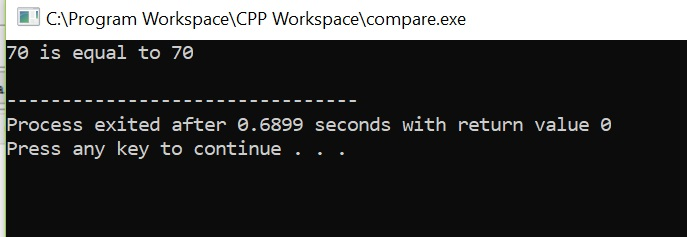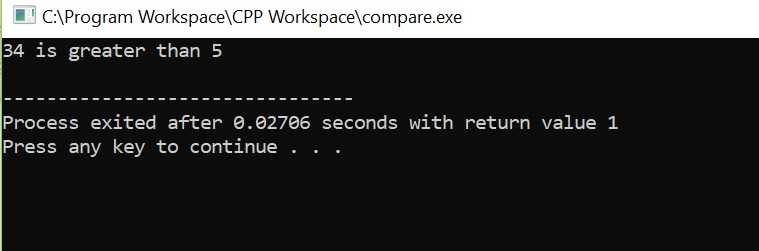# Write a program in C or a script in bash, called “compare” that takes two numbers on the command line and compares them....

Write a program in C or a script in bash, called “compare” that takes two numbers on the command line and compares them. The program should print the result of the comparison. Specifically, it should print “<x> is <comparison> <y>”, where <x> is the first number, <y> is the second number and <comparison> is one of “equal to”, “greater than” or “less than”. If the two numbers are equal, the program should have an exit status of zero. The exit status should be non-zero in all other cases. Use pseudo-code if necessary, but try to use valid C or bash syntax wherever possible. You may write the program and test it on UNIX. Write or copy/paste your code here.

 C Program ```#include int main(int numargs, char **argv) { // check if the number of command line arguments is 3 or not if(numargs == 3) { // convert the arguments to integers int x = atoi(argv); int y = atoi(argv); // compare the numbers if( x > y ) printf("%d is greater than %d\n", x, y ); else if(y > x) printf("%d is less than %d\n", x, y ); // else they are equal else { printf("%d is equal to %d\n", x, y ); return 0; } } // outer else else printf("Invalid number of arguments supplied!"); return 1; }```

OUTPUT##### Add Answer of: Write a program in C or a script in bash, called “compare” that takes two numbers on the command line and compares them....
More Homework Help Questions Additional questions in this topic.